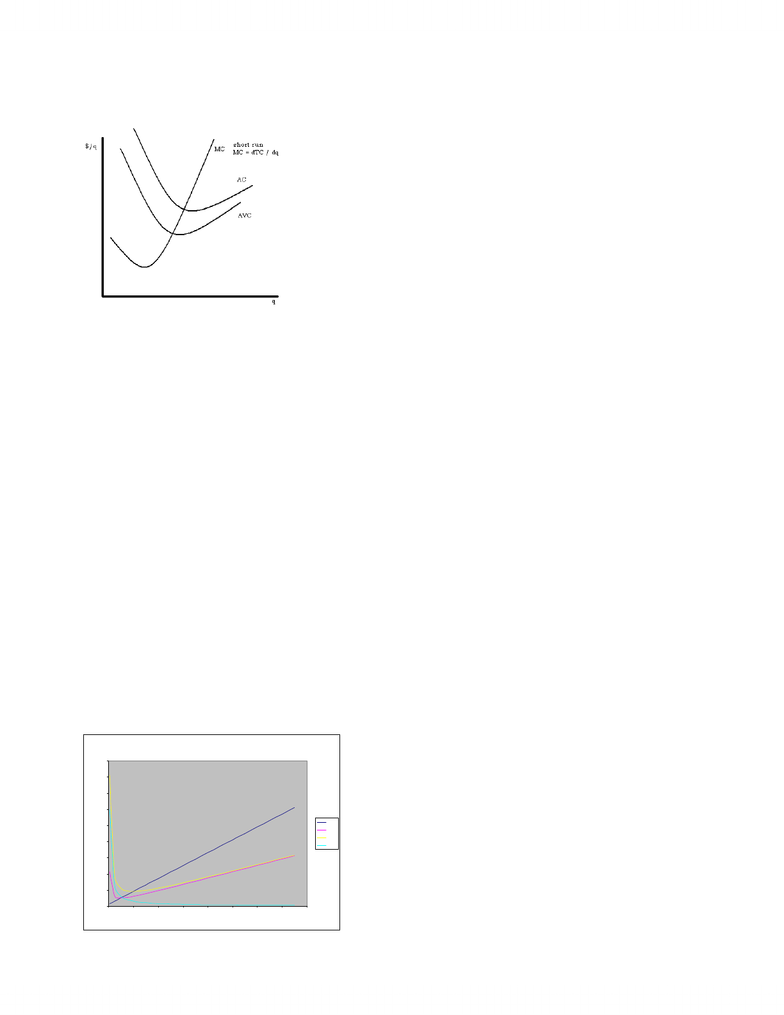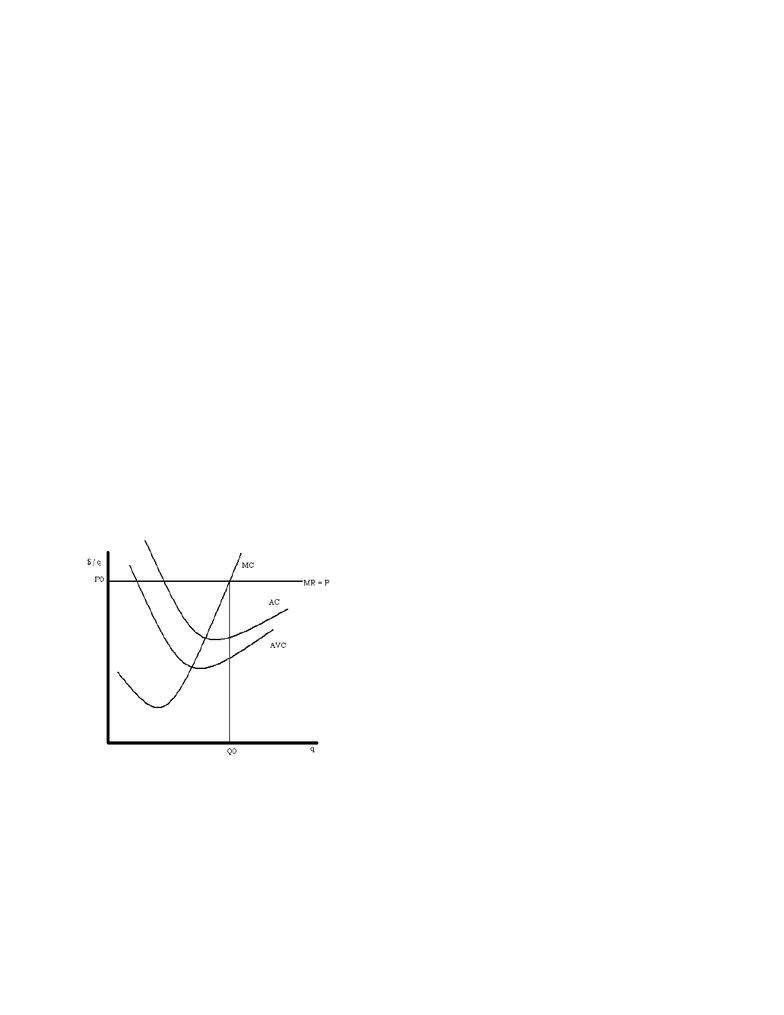Class Notes (1,100,000)
CA (640,000)
UTSC (30,000)
MGEA02H3 (200)
Lecture 6

# Lecture notes week 6

Department
Economics for Management Studies
Course Code
MGEA02H3
Professor
Gordon Cleveland
Lecture
6

This preview shows page 1. to view the full 5 pages of the document.ECMA04H Week 6
Costs: how do they behave as output increases?
What does the typical graph of cost curves look like (in the short run)?
What is the algebra of cost curves?
Example: TC = 400 + 5q + q2
FC = 300
VC = 100 + 5q + q2
MC = dTC / dq = dVC / dq = 5 + 2q
Rate of change of firm’s costs as output increases; incremental cost
Without calculus…./, MC = 'TC/'q (an approximation)
AC = TC / q = (400 + 5q + q2) / q = 400 / q + 5 + q
AC = (FC + VC)/q = AFC + AVC
AFC = 300 / q
AVC = (100 + 5q + q2) / q = 100 / q + 5 + q
AC = 300 / q + 100 / q + 5 + q
Find minimum of AC, AVC
AC = 400 / q + 5 + q
dAC / dq = -400 / q2 + 1 = 0, which implies that q = 20
AC = 400 / 20 + 5 + q = 20 + 5 + 20 = 45
At an output level of 20, AC will reach its minimum at a cost of \$45.
AVC = 100 / q + 5 + q
dAVC / dq = -100 / q2 + 1 = 0, which implies that q = 10
AVC = 100 / 10 + 5 + 10 = 10 + 5 + 10 = 25
At an output level of 10, AVC will reach its minimum at a cost of \$25.
Check value of MC at min AC and min AVC
MC = 5 + 2q
At q = 20, for AC, MC = 5 + 2 * 20 = \$45
At q = 10 for AVC, MC = 5 + 2 * 10 = \$25
Graph MC, AC, AVC
Cost Graph
0
50
100
150
200
250
300
350
400
450
0 20 40 60 80 100 120 140 160
q
\$ / q
MC
AVC
AC
AFC
www.notesolution.com

Unlock to view full version

Only page 1 are available for preview. Some parts have been intentionally blurred.How will these costs affect profit maximization by the individual firm?
We assume that firm’s objective is to maximize profit (which is represented by pi)
3 (pi) = TR (total revenue) – TC (total costs) = Pq – TC function
Notice that this must include all costs
Example: video store operated by owner.
Every week: \$2500 in rental income, pay out \$1000 for rental of space, \$800 for new videos, no pay for owner, but the OC of his
labour is \$500 per week and the normal return on his money invested is \$200.
Accounting profit = \$700 = 2500 – 1000 – 800 (explicit costs only)
Economic profit = \$0 = 2500 – 1000 – 800 – 500 – 200 (implicit costs included)
Economic profit must include costs of all resources used by the business that have alternative opportunities (i.e., the labour of the
owner and family, perhaps interest on owner-invested capital)
In general Accounting profit > Economic profit
Zero economic profit generally means positive accounting profits.
Back to my question:
How will these costs affect profit maximization by the individual firm?
3 = TR TC
But TR is a function of q and TC is a function of q.
To max: d3/dq = dTR/dq – dTC/dq = MR (marginal revenue) – MC (marginal cost)
Set = 0, so MR – MC = 0 or MR = MC maximizes profit
We know what MC is, what about MR?
Let’s imagine that we had a very small firm with no market power. This firm has no influence over general market price of the good it
is selling. All it can do is accept the “going price” and try to sell as much as it is sensible to sell.
To this firm, P is a constant.
Then, since TR = P x q, dTR / dq = P (the firm’s marginal revenue is equal to P—which is a constant)
For example, the going price could be \$25 per unit (P = MR = \$25) or \$45 per unit (P = MR = \$45) or \$65 per unit (P = MR = \$65)
Then the rule for maximizing profit is to find the quantity of output at which MC = P (i.e., choose the quantity at which P = MC). This
will be the profit-maximizing amount of output to produce.
Graph:
We have been talking about short run costs and short run profit maximization. What about long-run costs of the individual business
firm?
In LR, choose optimal amount of capital (all choices open).
Each SRAC (short – run AC) (or SAC) is associated with one level of capital
“Envelope”
www.notesolution.com